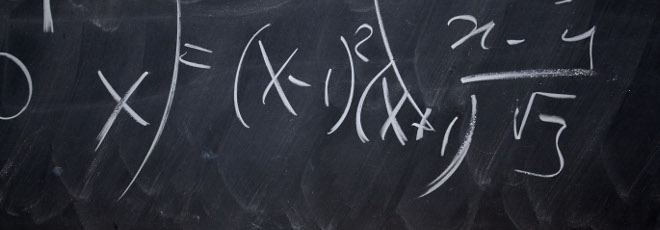## “On the compensator of step processes in progressively enlarged filtrations and related control problems”

Venerdì 24 Febbraio 2023, ore 14:30 - Aula 2BC60 - Paolo Di Tella (TU Dresda)

Abstract

For a step process $X$ with respect to its natural filtration $\mathbb F$, we denote by $\mathbb G$ the smallest right-continuous filtration containing $\mathbb G$ and such that another step process $H$ is adapted. We investigate some structural properties of the step process $X$ in $\mathbb G$: We show that $Z=(X,H)$ possesses the weak representation property with respect to $\mathbb G$ and, in the case $H=1_{[\tau,+\infty)}$, where $\tau$ is a random time (but not an $\mathbb F$-stopping time) satisfying Jacod’s absolute continuity hypothesis, we compute the $\mathbb G$-predictable compensator $\nu^{\mathbb G,X}$ of the jump measure of $X$.

Thanks to our theoretical results on $\nu^{\mathbb G,X}$, we can consider stochastic control problems related to model uncertainty on the intensity measure of $X$, also in presence of an external risk source modeled by the random time $\tau$. As a particular example we study a problem of exponential utility maximization for defaultable claims under a worst-case scenario.

This is a joint work with Elena Bandini (Uni Bologna) and Fulvia Confortola (PoliMi)